#### Homogeneous transformation matrices for 2D chains

We are now prepared to determine the location of each link. The location in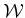of a point in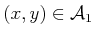is determined by applying the 2D homogeneous transformation matrix (3.35),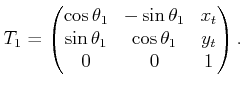(3.51)

As shown in Figure 3.10, let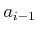be the distance between the joints in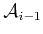. The orientation difference between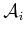andis denoted by the angle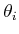. Let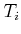represent a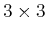homogeneous transformation matrix (3.35), specialized for linkfor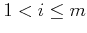,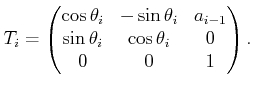(3.52)

This generates the following sequence of transformations:
1. Rotate counterclockwise by.
2. Translate byalong the-axis.
The transformationexpresses the difference between the body frame ofand the body frame of. The application ofmovesfrom its body frame to the body frame of. The application of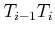moves bothandto the body frame of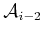. By following this procedure, the location inof any point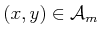is determined by multiplying the transformation matrices to obtain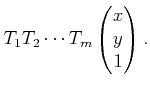(3.53)

Example 3..3 (A 2D Chain of Three Links)   To gain an intuitive understanding of these transformations, consider determining the configuration for link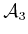, as shown in Figure 3.11. Figure 3.11a shows a three-link chain in which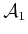is at its initial configuration and the other links are each offset by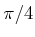from the previous link. Figure 3.11b shows the frame in which the model foris initially defined. The application of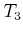causes a rotation of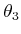and a translation by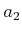. As shown in Figure 3.11c, this placesin its appropriate configuration. Note that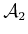can be placed in its initial configuration, and it will be attached correctly to. The application of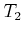to the previous result places bothandin their proper configurations, andcan be placed in its initial configuration.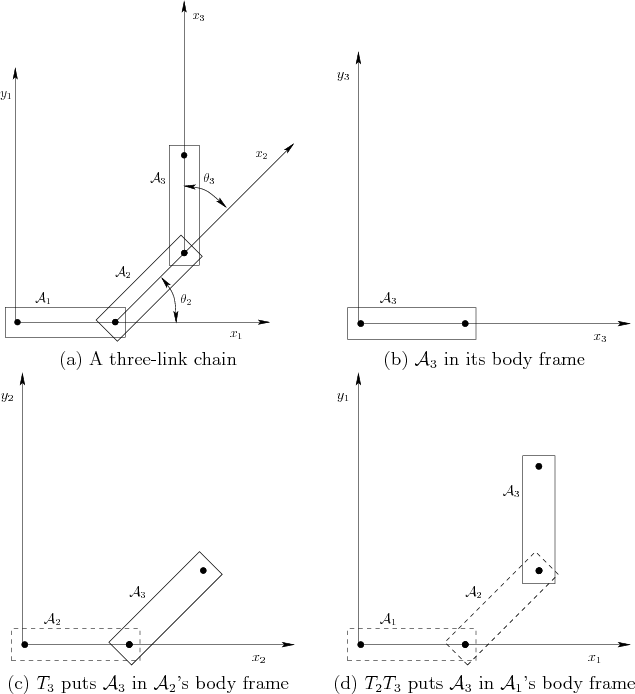For revolute joints, the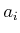parameters are constants, and theparameters are variables. The transformed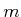th link is represented as. In some cases, the first link might have a fixed location in the world. In this case, the revolute joints account for all degrees of freedom, yielding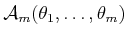. For prismatic joints, theparameters are variables, instead of theparameters. It is straightforward to include both types of joints in the same kinematic chain.

Steven M LaValle 2020-08-14